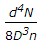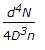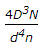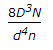# Civil Engineering - Theory of Structures - Discussion

### Discussion :: Theory of Structures - Section 1 (Q.No.25)

25.

The stiffness of the close coil helical spring is

 [A].[B].[C].[D].Explanation:

No answer description available for this question.

 P.Mohan said: (Aug 9, 2015) Explain how to derive it?

 Mohan Kumar said: (Jan 27, 2017) We know deflection of spring= 64 wR^3N/cd^4. Stiffness = load/ deflection. So, subtitute R=D/2 & calculate stiffness, we get cd^4/8D^3n.

 Murali said: (Aug 25, 2017) Explain the terms.

 Bairu Ganesh said: (Oct 14, 2021) Deflection= 64w (r^3) n/Nd^4 where stiffness= load/deflection load p = w. We know that radius r = D/2 and substitute i.e. deflection = 64w (D/2) ^3n/Nd^4=64wD^3n/8Nd^4. = 8wD^3n/Nd^4. Now stiffness = load/deflection = w/ (8wD^3n/Nd^4) = nd^4N/8D^3n.